# REASONING (Test 2)

Tag: reasoning
Q.1
Which is the odd one out?
A.B.C.D.Explaination / Solution:

It has three white and one black on the left and three black and one white on the right. The rest are the opposite way round.

Workspace
Report
Q.2
A person travels 12 km due North, then 15 km due East, after that 15 km due West and then 15 km due South. How far is he from the starting points?
A. 6 km
B. 12 km
C. 33 km
D. 60 km
Explaination / Solution:
No Explaination.

Workspace
Report
Q.3
P, Q, R and S are four men. P is the oldest but not the poorest. R is the richest but not the oldest. Q is older than S but not than P or R. P is richer than Q but not than S. The four men can be ordered (descending) in respect of age and richness, respectively, as:
A. PQRS, RPSQ
B. PRQS, RSPQ
C. PRQS, RSQP
D. PRSQ, RSPQ
Explaination / Solution:
No Explaination.

Workspace
Report
Q.4
If Sohan, while selling two goats at the same price, makes a profit of 10% on one goat and suffers a loss of 10% on the other
A. he makes no profit and no loss.
B. he makes a profit of 1%.
C. he suffers a loss of 1%.
D. he suffers a loss of 2%.
Explaination / Solution:
No Explaination.

Workspace
Report
Q.5

Consider that:

1. A is taller than B.
2. C is taller than A.
3. D is taller than C.
4. E is the tallest of all.

If they are made to sit in the above order of their height, who will occupy the mid position?

A. A
B. B
C. C
D. D
Explaination / Solution:
No Explaination.

Workspace
Report
Q.6
Find the minimum number of straight lines required to make the given figure.A. 16
B. 17
C. 18
D. 19
Explaination / Solution:

The figure may be labelled as shown.The horizontal lines are IK, AB, HG and DC i.e. 4 in number.

The vertical lines are AD, EH, JM, FG and BC i.e. 5 in number.

The slanting lines are IE, JE, JF, KF, DE, DH, FC and GC i.e. 8 is number.

Thus, there are 4 + 5 + 8 = 17 straight lines in the figure.

Workspace
Report
Q.7
Find the number of triangles in the given figure.A. 10
B. 19
C. 21
D. 23
Explaination / Solution:

The figure may be labelled as shown.The simplest triangles are ABI, BIC, AIJ, CIJ, AHJ, CDJ, JHG, JDE, GJF and EJF i.e. 10 in number.

The triangles composed of two components each are ABC, BCJ, ACJ, BAJ, AJG, CJE and GJE i.e. 7 in number.

The triangles composed of four components each are ACG, ACE, CGE and AGE i.e. 4 in number.

Total number of triangles in the figure =10+ 7 + 4 = 21.

Workspace
Report
Q.8

Find the number of triangles in the given figure.A. 18
B. 20
C. 28
D. 34
Explaination / Solution:

The figure may be labelled as shown.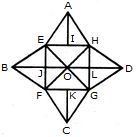The simplest triangles are AEI, AIH, BEJ, BJF, CFK, CKG, DGL, DLH, EOJ, FOJ, FOG, LOG, HOL and HOE i.e. 14 in number.

The triangles composed of two components each are EAH, FBE, BEO, EOF, BFO, FCG, GDH, HOD, HOG and GOD i.e. 10 in number.

The triangles composed of three components each are EFH, EHG, FGH and EFG i.e. 4 in number.

Thus, there are 14 + 10 + 4 = 28 triangles in the given figure.

Workspace
Report
Q.9

Count the number of squares in the given figure.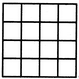A. 32
B. 30
C. 29
D. 28
Explaination / Solution:

The figure may be labelled as shown.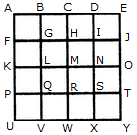The simplest squares are ABGF, BCHG, CDIH, DEJI, FGLK, GHML, HINM, IJON, KLQP, LMRQ, MNSR, NOTS, PQVU, QRWV, RSXW and STYX i.e. 16 in number.

The squares composed of four components each are ACMK, BDNL, CEOM, FHRP, GISQ, HJTR, KMWU, LNXV and MOYW i.e. 9 in number.

The squares composed of nine components each are ADSP, BETQ, FIXU and GJYV i.e. 4 in number.

There is one square AEYU composed of sixteen components.

There are 16 + 9 + 4 + 1 = 30 squares in the given figure.

Workspace
Report
Q.10

Count the number of triangles and squares in the given figure.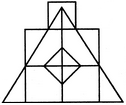A. 21 triangles, 7 squares
B. 18 triangles, 8 squares
C. 22 triangles, 7 squares
D. 20 triangles, 8 squares
Explaination / Solution:

The figure may be labelled as shown.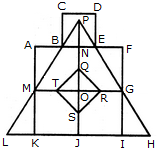Triangles :

The simplest triangles are BPN, PNE, ABM, EFG, MLK, GHI, QRO, RSO, STO and QTO i.e. 10 in number.

The triangles composed of two components each are BPE, TQR, QRS, RST and STQ i.e. 5 in number.

The triangles composed of three components each are MPO and GPO i.e. 2 in number.

The triangles composed of six components each are LPJ, HPJ and MPG i.e. 3 in number.

There is only one triangle LPH composed of twelve components.

Total number of triangles in the figure = 10 + 5 - 2 + 3 + 1 = 21.

Squares :

The squares composed of two components each are KJOM and JIGQ i.e. 2 in number.

The squares composed of three components each are ANOM, NFGO and CDEB i.e.3 in number.

There is only one square i.e. QRST composed of four components.

There is only one square i.e. AFIK composed of ten components.

Total number of squares in the figure = 2 + 3 + 1 + 1 = 7.

Workspace
Report